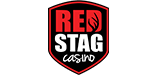# Play Sic BoYou may place multiple bets at the same time. You can identify the different bet areas on the table by placing the cursor on an area and reading the info text on the status bar (the low edge of the screen). Sic Bo features the following betting options:

## Small/Big Bet:

Status bar info text on these areas is “Small” or “Big”. Small Bet wins if the total sum of the three dice is 4 to 10. Big Bet wins on a total of 11 to 18. Neither Small nor Big Bet wins if the three dice are of the same number (three of a kind). The Small and Big Bet payoff ratio is 1 to 1.

## Number Bet:

Placing a number bet means you want a specific number to appear on as many dice as possible. Select a number from 1 to 6 and place your chip(s) on the corresponding area. Status bar info text for these areas is “One”, “Two”, “Three”, etc. If the chosen number appears 1 time your bet pays 1 to 1, if it appears 2 times your bet pays 2 to 1 and if it appears 3 to 1 your bet pays 3 to 1.

## Pair Bet:

Make a bet on any of the 15 possible two dice combinations (for example 3 and 4; 2 and 5 or 2 and 6). Status bar info text for these bets is “Pair”. If both numbers appear, your bet pays 5 to 1.

## Total Bet:

Placing a total bet means you want the total sum of the three dice to be a certain amount. Possible totals are from 4 to 17. 3 and 18 (which are also possible with three dice) are excluded. Status bar info text for these bet areas is “Total of 4”, “Total of 5”, “Total of 6”, etc. The pay ratios for the total bet depends on the sum:
 Total of 4 60 to 1 Total of 5 30 to 1 Total of 6 17 to 1 Total of 7 12 to 1 Total of 8 8 to 1 Total of 9 6 to 1 Total of 10 6 to 1 Total of 11 6 to 1 Total of 12 6 to 1 Total of 13 8 to 1 Total of 14 12 to 1 Total of 15 17 to 1 Total of 16 30 to 1 Total of 17 60 to 1

## Double Bet:

You bet on a specific pair to appear (for example, two 3’s). Status bar info text for these areas is “Double One”, “Double Two”, “Double Three”, etc. If at least two of the number chosen appear, you win 10 to 1.

## Triple Bet:

You bet on a specific triple to appear (for example, three 2’s). Status bar info text for there areas is “Triple One”, “Triple Two”, “Triple Three”, etc. If the chosen triple appears, you win 180 to 1.

## Any Triple:

You win if all three dice have the same number (any three of a kind). Status bar info text for this area is “Any Triple”. Payoff ratio for this bet is 30 to 1.